# Modeling, Forecasting and Simulating Commodity Prices through Term Structure Estimation using Kalman Filtering: The R Package ‘NFCP’

library(NFCP)

# Set seed for reproducibility:
set.seed(412)

# 1. Introduction

‘NFCP’ (N-Factor Commodity Pricing) provides a framework for the modeling, parameter estimation, probabilistic forecasting, European and American option valuation and simulation of commodity prices through state space and Monte Carlo methods, risk-neutral valuation and Kalman filtering. Commodity pricing models are (systems of) stochastic differential equations that are utilized for the valuation and hedging of commodity contingent claims (i.e. derivative products on the commodity) and other commodity related investments. Parameters of commodity pricing models are estimated through MLE, using available term structure futures data of a commodity. Commodity pricing models that capture market dynamics are of great importance to commodity market participants in order to exercise sound investment and risk-management strategies. The N-factor commodity pricing model framework was first presented in the work of Cortazar and Naranjo (2006) titled “An N-Factor Gaussian Model of Oil Futures Prices”.

The purpose of this vignette is to provide worked examples of the estimation and analysis of commodity pricing models using the N-factor framework of the ‘NFCP’ package. Examples presented within this vignette, as well as throughout the documentation of the ‘NFCP’ package, replicate the prolific work of Schwartz and Smith (2000) titled: “Short-Term Variations and Long-Term Dynamics in Commodity Prices”. The two-factor model presented within this study is replicated, with the primary figures and results replicated as well as discussion on how to interpret and analyze N-factor models. The remainder of this vignette is structured as follows. Section 2 provides a background of modeling commodity prices through term structure estimation. Section 3 introduces the N-factor stochastic modeling framework. Section 4 provides worked examples on the estimation and analysis of commodity pricing models, using the crude oil data first presented within the work of Schwartz and Smith (2000), replicating many of the results of this study. Section 5 provides a discussion of the N-factor commodity pricing model, the ‘NFCP’ package and concludes.

# 2. Term Structure Estimation

Modeling commodity prices is typically performed through term structure estimation, as futures markets provide valuable information regarding expectations of future price movements and other dynamics. Parameter estimation of commodity pricing models involves expressing the futures curve in terms of unobserved factors and deriving analytic expressions for futures prices under no-arbitrage conditions. Term structure estimation of commodity pricing models was popularized within the works of Gibson and Schwartz (1990), Schwartz (1997, 1998) and Schwartz and Smith (2000). These commodity pricing models make differing assumptions regarding the innovation, mean-reverting behavior, consideration of the cost-of-carry, and the number of correlated factors to explain the term structure of commodities. These commodity pricing models (or their affine transformation equivalents, see Cortazar and Naranjo (2006)) were subsequently unified under the N-factor framework of Cortazar and Naranjo (2006), expressing the log of the commodity spot price processes as the sum of N correlated factors. ‘NFCP’ considers commodity pricing models under this N-factor framework.

Kalman filtering is the process of obtaining unreliable, but measurable, observations and estimating a time series of unobservable measurements in return, with an allowable degree of measurement error. Kalman filtering is used to estimate parameters of commodity pricing models through MLE (Gibson and Schwartz (1990), Schwartz (1997, 1998), Schwartz and Smith (2000), Cortazar and Naranjo (2006), Aspinall et al. (2020). There exist several implementations of the Kalman filtering algorithm within existing R packages (of which Tussell (2011) provides a review), with the most recent being the ‘FKF.SP’ package. Applying Kalman filtering to the term structure of commodities requires the algorithm to allow for intercepts and for missing data, as is characteristic of futures markets, as not every contract is traded every day and contracts expire or become available over a given observation period. An assumption taken by existing literature and the N-factor model is that the variance of the measurement equation (i.e. the ‘white noise’ of the observed contract error) is independent (or identical), which allows for the application of sequential processing. Sequential processing is a univariate treatment of a multivariate series of observations and can benefit from computational efficiency over traditional Kalman filtering (Durbin and Koopman, 2001; Aspinall et al., 2021). The ‘NFCP’ package performs Kalman filtering through the ‘FKF.SP’ package (Aspinall et al., 2021).

Parameter estimation of commodity pricing models in ‘NFCP’ is performed through Kalman filtering and MLE. Parameter estimation of N-factor models features many unknown parameters, a large number of observations and an objective function (the log-likelihood) that is discontinuous and nonlinear, with multiple local optima. The complexity of parameter estimation increases substantially according to the number of factors in the model. The number of unknown parameters of an N-factor model (without considering the white noise of futures contracts) is equal to: $$0.5 (N^2 + 5N)$$. MLE is performed using genetic algorithms through the ‘genoud’ function of the ‘rgenoud’ package to maximize the likelihood of obtaining MLE values.

## Section 3: The N-Factor Model

The N-factor commodity pricing model was first presented in the work of Cortazar and Naranjo (2006, equations 1-3). Following the work of Cortazar and Naranjo (2006), the N-factor framework describes the spot price process of a commodity as the correlated sum of $$N$$ state variables $$x_{t}$$.

$log(S_{t}) = \begin{cases} \sum_{i=1}^N x_{i,t} &\text{GBM = T} \\ E+\sum_{i=1}^N x_{i,t} &\text{GBM = F} \\ \end{cases}$

where state variables (i.e. factors of the spot price) follow correlated mean-reverting (i.e. Ornstein-Uhlenbeck) processes, with the first state variable further able to be considered to follow a random walk (i.e. Brownian Motion process) to induce a unit root in the spot price process when logical argument $$GBM$$ is true. The valuation of derivative products and commodity-related investments requires the “risk-neutral” version of the model. Here (and below) asterisks are used to denote the risk-neutral versions of variables and processes rather than the “true” process. Under the assumption of risk-neutrality the state variables follow the process:

$dx_{1,t} = \begin{cases} \mu^*dt + \sigma_{1} dw_{1}t &\text{GBM = T} \\ - (\lambda_{1} + \kappa_{1}x_{1,t})dt + \sigma_{1} dw_{1}t &\text{GBM = F} \\ \end{cases}$

$dx_{i,t} =_{i\neq 1} - (\lambda_{i} + \kappa_{i}x_{i,t})dt + \sigma_{i} dw_{i}t$

Where: $E(w_{i})E(w_{j}) = \rho_{i,j}$

The following constants are defined as:

$$\mu$$: long-term growth rate

$$E$$: Constant equilibrium level

$$\kappa_{i}$$: Reversion rate of state variable $$i$$

$$\sigma_{i}$$: the instantaneous volatility of state variable $$i$$

$$\rho_{i,j} \in [-1,1]$$: the instantaneous correlation between state variables $$i$$ and $$j$$

The risk-neutral variables are defined as:

$$\mu^*=\mu-\lambda_1$$: Long-term risk-neutral growth rate

$$\lambda_{i}$$: Risk premium of state variable $$i$$

Under the assumption of risk-neutrality, state variables with mean-reverting behaviour revert towards the equilibrium value $$- \frac{\lambda_{i}}{\kappa_{i}}$$ at a rate of $$\kappa_{i}$$ with a respective half-life of $$\frac{log(2)}{\kappa_{i}}$$.

Under the N-factor model, the state variables under the risk-neutral process are jointly distributed with mean vector and covariance matrix:

$E^*[x_i(t)] = \begin{cases} \ x_i(0) + \mu^* t &i=1 \text{ and GBM=T} \\ \ x_i(0)e^{-(\kappa_i t)} - \frac{(1-e^{-\kappa_it})\lambda_i}{\kappa_i} &\text{otherwise} \\ \end{cases}$ and:

$Cov^*_{i,j}(x_{i,t},x_{j,t}) = Cov_{i,j}(x_{i,t},x_{j,t}) = \begin{cases} \sigma_1^2(t) &i=1,j=1 \text{ and GBM = T} \\ \sigma_i\sigma_j\rho_{i,j}\frac{1-e^{-(\kappa_i+\kappa_j)t}}{\kappa_i+\kappa_j} &\text{otherwise} \\ \end{cases}$

Filtering the N-factor model through the Kalman filter, is described in ‘help(NFCP.Kalman.filter)’.

## Section 4: Using the ‘NFCP’ package:

The following section details how to replicate major findings, plots and tables from the prolific work of Schwartz and Smith (2000). The Schwartz and Smith (2000) two-factor model is one of the most popular commodity pricing models. Schwartz and Smith (2000) presented a two-factor model, where the first model followed a brownian motion, and applied it to WTI Crude Oil data from 1990 - 1995. The approximate crude oil data, as well as estimated parameters and modeling assumptions, used within the original work of Schwartz and Smith (2000) is included within ‘NFCP’ within the SS.Oil list object. See help(SS.Oil) for more details.

The parameters of the Schwartz and Smith two-factor model are:

SS.Oil.Two.Factor.parameters <- NFCP.Parameters(N.factors = 2,
GBM = TRUE,
Initial.State = FALSE,
S.Constant = FALSE,
N.contracts = 5)
#>
#> ----------------------------------------------------------------
#> 2 Factors model: first factor is a GBM
#>
#> Risk Neutral SDE:
#>
#> log(S_t) = sum(x_t)
#>
#> Where:
#> d x1_t    = mu_star * dt  + sigma_1 * dW_1
#> d x2_t    = - (lambda_2 + kappa_2 * x2_t) * dt  + sigma_2 * dW_2
#>
#>  And:
#>
#> E(dW_1 * dW_2) = rho_1_2 * dt
## Print the vector of parameters of the specified commodity pricing model:
print(SS.Oil.Two.Factor.parameters)
#>   "mu"               "mu_star"          "lambda_2"         "kappa_2"
#>   "sigma_1"          "sigma_2"          "rho_1_2"          "sigma.contract_1"
#>   "sigma.contract_2" "sigma.contract_3" "sigma.contract_4" "sigma.contract_5"

### 4.1 Stitching Futures Data

Traditional implementations of the Kalman filter to term structure data stitches contracts together according to their ordering by maturity. This results in a dataset where “for all given dates in the estimation sample, prices for the same set of contracts (with the same maturities) (are observed)” (Cortazar and Naranjo, 2006). Contracts have been stitched within existing literature in order to attain a dataset that has no missing observations and a time homogeneous time-to-maturity of observations - resulting in the Kalman filter quickly approaching a steady state distribution. Contracts are rolled over as the closest contract expires. Aggregating futures data creates information loss due to discrepancies between the actual time-to-maturity of observations and the assumed, time homogeneous time-to-maturity. Information loss also occurs as not all available contracts at a given point in time are observed within the model. Studies that have developed commodity pricing models on stitched data include: Longstaff and Schwartz (1990), Schwartz (1997), Schwartz and Smith (2000), Aspinall et al., (2020).

The approximate stitched data used to estimate commodity pricing models in the work of Schwartz and Smith (2000) is provided within the SS.Oil list object. Stitching futures contracts to obtain a complete panel dataset can be developed through the ‘Stitch.Contracts’ function.

###Method 1 - Stitch Crude Oil Contracts according to maturity matching:
SSOilStitched <- Stitch.Contracts(Futures = SS.Oil$Contracts, TTM = c(1, 5, 9, 13, 17)/12, maturity.matrix = SS.Oil$Contract.Maturities,
rollover.frequency = 1/12, verbose = TRUE)

##Plot the Stitched Maturities:
matplot(as.Date(rownames(SSOilStitched$Maturities)), SSOilStitched$Maturities,
type = 'l', main = "Stitched Contract Maturities",
ylab = "Time To Maturity (Years)", xlab = "Date", col = 1)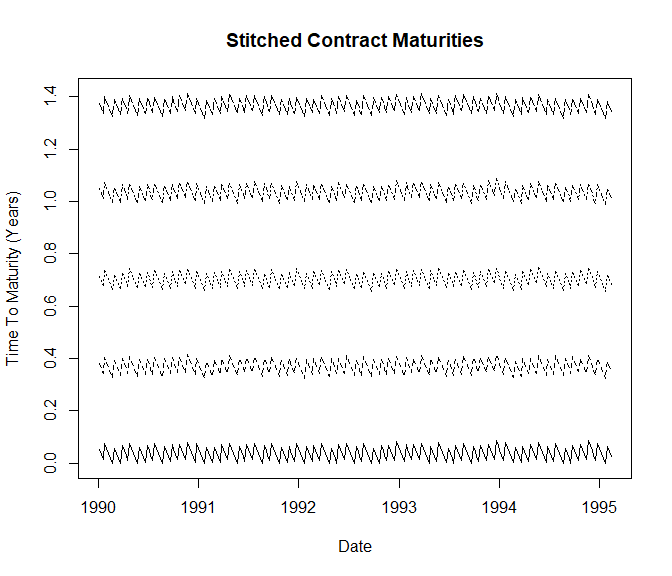The plot above is highly similar to Figure 1. of Schwartz (1997), although for a different observation period and different fixed maturities of stitched contracts.

Rather than stitching futures contracts to obtain a complete panel dataset, several other studies have instead used incomplete panel data of all available futures contracts to ensure there is no information loss during MLE (Sørensen, 2002; Cortazar and Schwartz, 2003; Cortazar and Naranjo, 2006). For the Schwartz and Smith (2000) oil contract data, there are an average of 21 available contracts at each observation. Both complete and incomplete panel data is compatible throughout the ‘NFCP’ package.

Whilst stitching data does result in information loss, the increase in relative algorithm efficiency compared to contract data can make this data structure appealing, particularly for estimating commodity pricing models with several state variables. Stitched futures data can also consider the white noise of each stitched contract during parameter estimation, which can provide a greater fit to the commodity pricing model. A commodity pricing model that considers all available contracts at a given point in time instead takes the assumption that the white noise of each futures contract is independent and identical (as was assumed in the work of Cortazar and Naranjo (2006)).

### 4.2 Filtering commodity pricing models:

The parameters estimated by Schwartz and Smith (2000) are available within the ‘SS.Oil’ list object as object ‘Two.Factor’. Named vectors of parameters such as the one shown below are used throughout the ‘NFCP’ package for filtering, forecasting and simulating particular estimated models.

print(SS.Oil$Two.Factor) #> mu mu_star lambda_2 kappa_2 #> -0.0125 0.0115 0.1570 1.4900 #> sigma_1 sigma_2 rho_1_2 sigma.contract_1 #> 0.1450 0.2860 0.3000 0.0420 #> sigma.contract_2 sigma.contract_3 sigma.contract_4 sigma.contract_5 #> 0.0060 0.0030 0.0000 0.0040 #### 4.2.1 Complete, Stitched Data: Estimated parameters of N-factor models can be filtered using the Kalman filter through the ‘NFCP.Kalman.filter’ function to obtain filtered values of the factors, as well as other measures of model fit and robustness. ##Example 1 - Replicating the Schwartz and Smith (2000) ##Two-Factor Crude Oil commodity pricing model: SS.Oil.2F <- NFCP.Kalman.filter( parameter.values = SS.Oil$Two.Factor,
parameters = names(SS.Oil$Two.Factor), log.futures = log(SS.Oil$Stitched.Futures),
TTM = SS.Oil$Stitched.TTM, dt = SS.Oil$dt,
verbose = TRUE,
debugging = TRUE)

It’s important to note that when ‘verbose = TRUE’, the Kalman filter iteration used by the ‘NFCP.Kalman.filter’ function does not use sequential processing and is a slower Kalman filter process not suited for parameter estimation.

#### 4.2.2 All Available Contracts, Incomplete Data:

The Kalman filter can be applied to all available futures contracts by passing the contract data and time to maturity of contracts at each observation into the ‘NFCP.Kalman.filter’ function. Applying the Kalman filter to all available contracts requires the assumption that the white noise of futures contracts is independent and identical:

### Assume constant white noise in parameters of 1%:
SS.Oil.Two.Factor <- SS.Oil$Two.Factor SS.Oil.Two.Factor <- SS.Oil.Two.Factor[!grepl("contract", names(SS.Oil.Two.Factor))] SS.Oil.Two.Factor["sigma.contracts"] <- 0.01 SS.Oil.2F.Contracts <- NFCP.Kalman.filter( parameter.values = SS.Oil.Two.Factor, parameters = names(SS.Oil.Two.Factor), log.futures = log(SS.Oil$Contracts),
TTM = SS.Oil$Contract.Maturities, dt = SS.Oil$dt,
verbose = TRUE,
debugging = TRUE)

### 4.3 Estimating N-Factor Commodity Pricing Models:

Parameter estimation of commodity pricing models is performed through the ‘NFCP.MLE’ function. The ‘NFCP.MLE’ function is a wrapper of the ‘NFCP.Kalman.filter’ function to the ‘genoud’ function of the ‘rgenoud’ package. The ‘genoud’ function uses genetic algorithms to solve optimization problems such as MLE (Mebane and Sekhon, 2011). All arguments of the ‘genoud’ function can be passed through the ‘NFCP.MLE’ function and can have a large impact on the estimated parameters of a commodity pricing model. Increasing the population size in particular can have a large impact on maximum likelihood estimates at the cost of increasing the computation time of the estimation process, with population sizes of up to 5-10,000 potentially increasing the performance of MLE for estimating complex commodity pricing models.

The following example presents an estimation of a one-factor Geometric Brownian Motion model to the Schwartz and Smith (2000) crude oil dataset. This function call can take up to one-minute to execute:

# Estimate a GBM model:
SS.Oil.1F <- NFCP.MLE(
## Arguments
log.futures = log(SS.Oil$Stitched.Futures), dt = SS.Oil$dt,
TTM= SS.Oil$Stitched.TTM, S.Constant = FALSE, N.factors = 1, GBM = TRUE, cluster = NULL, Richardsons.Extrapolation = TRUE, ## Genoud arguments: pop.size = 1000, optim.method = "L-BFGS-B", print.level = 0, hessian = TRUE, max.generations = 100, solution.tolerance = 0.1) #> #> ---------------------------------------------------------------- #> 1 Factor model: first factor is a GBM #> #> Risk Neutral SDE: #> #> log(S_t) = sum(x_t) #> #> Where: #> d x1_t = mu_star * dt + sigma_1 * dW_1 #> ---------------------------------------------------------------- #> Term Structure Estimation: #> 8 Unknown Parameters #> 268 Observations #> 5 Futures Contracts ##Print results: print(cbind(Estimated Parameters = round(SS.Oil.1F$Estimated.Parameters,4),
Standard Error = round(SS.Oil.1F$Standard.Errors,4))) #> Estimated Parameters Standard Error #> mu -0.0270 0.1024 #> mu_star -0.0336 0.0030 #> sigma_1 0.2299 0.0099 #> sigma.contract_1 0.0876 0.0038 #> sigma.contract_2 0.0326 0.0014 #> sigma.contract_3 0.0000 0.0019 #> sigma.contract_4 0.0186 0.0008 #> sigma.contract_5 0.0305 0.0013 ### 4.4 N-Factor Model Comparison: Log-likelihood scores of different N-factor models can be directly compared (given that they’ve been estimated using the same dataset) in order to test whether additional factors have had a significant improvement to the ability of the model to describe the term structure of the commodity. Consider the crude oil data of Schwartz and Smith (2000): The log-likelihood score of a GBM model estimated on this term structure data is: print(c(Log Likelihood: One-Factor = SS.Oil.1F$MLE))
#> Log Likelihood: One-Factor
#>                   2593.508

The log-likelihood score of the two-factor model presented by Schwartz and Smith (2000) is:

print(c(Log Likelihood: Two-Factor = SS.Oil.2F$LL)) #> Log Likelihood: Two-Factor #> 4018.632 The GBM model can be obtained from the two-factor model by considering uncertainty in factor 1 only (i.e. $$x_{(2,0)} ,\lambda_2, \sigma_2$$ are zero). The relevant test statistic for this comparison is a chi-squared test with 3 degrees of freedom with a 99th percentile of this distribution being 11.31 (Schwartz and Smith, 2000). In this particular case, the log-likelihood score of the two-factor model is much greater than that of the one-factor GBM, indicating that an additional factor has greatly improved the fit of the commodity pricing model. Similar statistical testing can be done for different N-factor models. ### 4.5 N-Factor Model Term Structure Fit: The errors in the model Fit to the logarithm of futures prices were presented in table 3 of Schwartz and Smith (2000). These errors, along with the root mean squared (RMS) error is returned by the ‘NFCP.Kalman.filter’ function: ##Replicate Table 3 of Schwartz and Smith (2000): print(round(SS.Oil.2F$TSFit.Error,4))
#>                         F1      F5     F9 F13    F17
#> Mean Error          0.0068 -0.0004 0.0002   0 0.0001
#> Mean Absolute Error 0.0318  0.0034 0.0021   0 0.0029
#> SD Error            0.0424  0.0043 0.0027   0 0.0037
#> RMSE                0.0429  0.0043 0.0027   0 0.0037

The estimated error for contract “F13” in the two-factor model presented by Schwartz and Smith (2000) was estimated to be zero, meaning the commodity pricing model perfectly matched futures prices of the contract at each observation point. The two-factor model is therefore most accurate for forecasting medium-term futures prices. The N-factor model is able to perfectly match up to N futures contracts during parameter estimation if the white noise of observations is not assumed identical (Schwartz and Smith, 2000).

Another measure of robustness is to evaluate the mean squared error (Bias) and root mean squared error (RMSE) of a commodity pricing model to the entire term structure dataset, rather than to individual contracts. The following is similar to table 3 presented by Cortazar and Naranjo (2006):

Bias <- c(One-Factor = SS.Oil.1F$Filtered.Error["Bias"], Two-Factor = SS.Oil.2F$Filtered.Error["Bias"])
RMSE <- c(One-Factor = SS.Oil.1F$Filtered.Error["RMSE"], Two-Factor = SS.Oil.2F$Filtered.Error["RMSE"])
print(round(cbind(RMSE, Bias), 4))
#>                   RMSE    Bias
#> One-Factor.RMSE 0.0448 -0.0041
#> Two-Factor.RMSE 0.0194  0.0013

The introduction of a second factor for this particular commodity pricing model has reduced total RMSE to the observed term structure dataset. It has also changed the model from being, on average, negatively biased to positively biased.

### 4.6 Plot Contract Observation Error:

The error in contracts at each observation point can be plot to provide an insight into which contracts are estimated with the highest level of error, and how the model fit changed over the observation period. These plots can be directly compared to visualise model fit of different N-factor models.

We begin by considering the contract observation error of the one-factor estimated GBM model:

##One Factor
matplot(as.Date(rownames(SS.Oil.1F$V)), SS.Oil.1F$V, type = 'l',
xlab = "", ylab = "Observation Error",
main = "Contract Observation Error: One-Factor Model"); legend("bottomright",
colnames(SS.Oil.2F$V),col=seq_len(5),cex=0.8,fill=seq_len(5))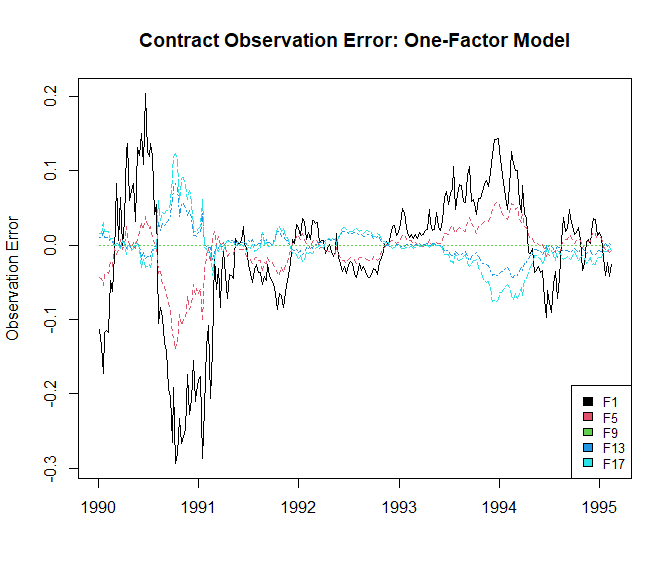##Two-Factor matplot(as.Date(rownames(SS.Oil.2F$V)), SS.Oil.2F$V, type = 'l', xlab = "", ylab = "Observation Error", ylim = c(-0.3, 0.2), main = "Contract Observation Error: Two-Factor Model"); legend("bottomright", colnames(SS.Oil.2F$V),col=seq_len(5),cex=0.8,fill=seq_len(5))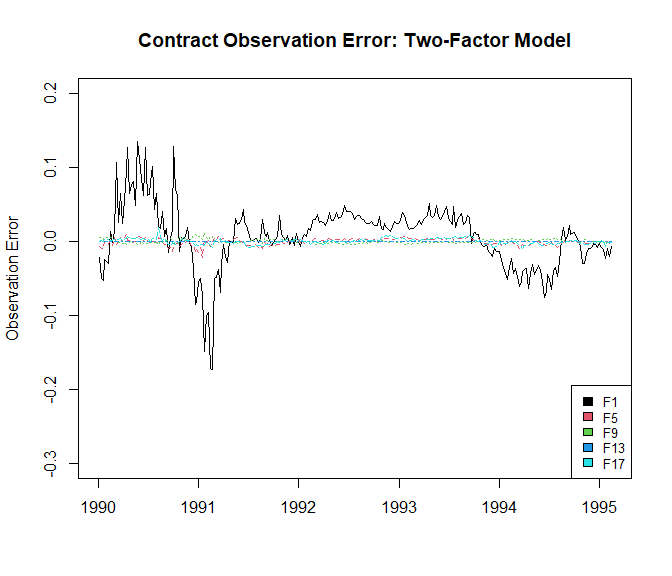Observation errors of the two-factor model were lower than the one-factor model in this case. For the two-factor model, observation error for the one month maturity contract is far higher than the other contracts, meaning the two-factor models ability to predict very short-term contracts is lower than middle and long term contracts.

Comparing this with the contract observation error of the estimated one-factor GBM model:

This is also shown by the root mean squared error (RMSE) of each contract:

matplot(cbind(SS.Oil.1F$TSFit.Error["RMSE",], SS.Oil.2F$TSFit.Error["RMSE",]),
type = 'l', main = "Root Mean Squared Error of Futures Contracts",
xlab = "Contract", ylab = "RMSE"); legend("right",c("One-Factor", "Two-Factor"),
col=1:2,cex=0.8,fill=1:2)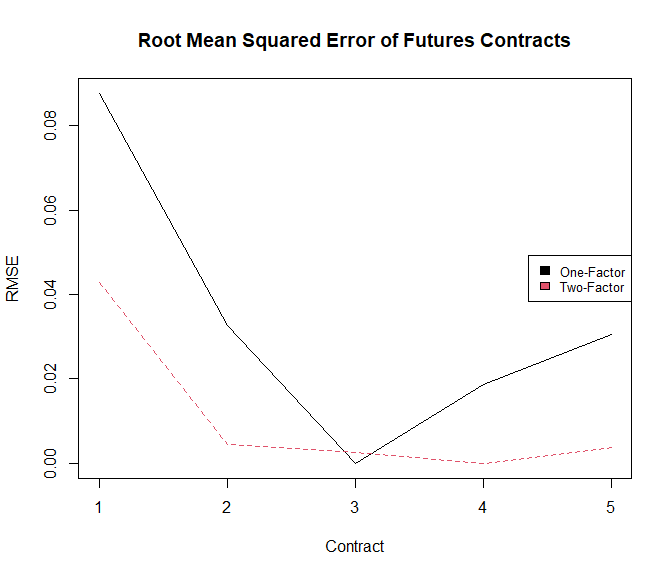The one-factor GBM model had the highest fit for the medium maturity contract, with higher errors for the very short- and long-term contracts, whilst the two-factor model had lower RMSE values overall, and less discrepancy between the RMSE values of the different contracts. These findings are consistent with those found by Aspinall et al. (2020) for the prices of emissions allowances in the European Union.

### 4.7 Plot filtered values:

The two-factor model can be interpreted as the decomposition of spot prices into short-term deviations (factor 2) and long-run equilibrium prices (factor 1). The estimated equilibrium and spot prices were presented in figure 4 of Schwartz and Smith (2000) and are replicated below:

##Replicate Figure 4 of Schwartz and Smith (2000):
SS.Figure.4 <- cbind(Equilibrium Price =
exp(SS.Oil.2F$X[,1]), Spot Price = SS.Oil.2F$Y[,"filtered Spot"])

matplot(as.Date(rownames(SS.Figure.4)), SS.Figure.4, type = 'l',
xlab = "", ylab = "Oil Price ($/bbl, WTI)", col = 1, main = "Estimated Spot and Equilibrium Prices for the Futures Data")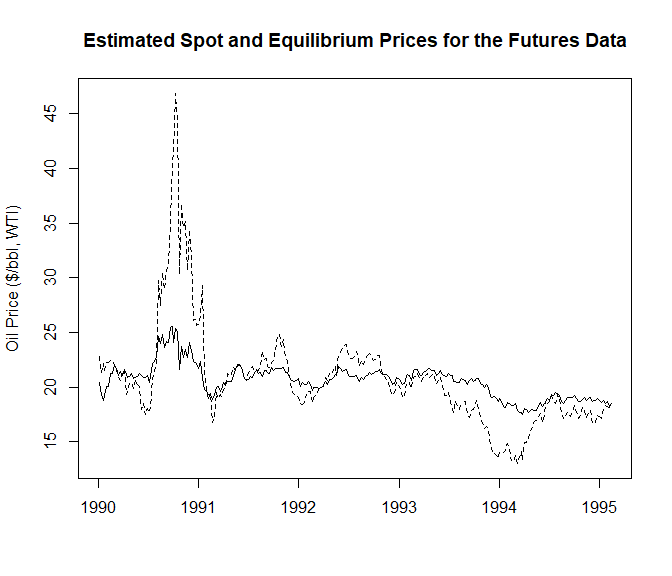### 4.8 Plot Standard Deviation of State Variables: Cortazar and Naranjo (2006) plot the time series of the standard deviation for state variables (Figures 2 & 3) for analysis of the behaviour of the state variables over the observation period. This can provide insights and complement analysis into exogenous shocks, structural breaks and the volatility of the factors that influence a commodity over time. Observation.Dates <- as.Date(rownames(SS.Oil$Contracts))

plot(Observation.Dates, sqrt(SS.Oil.2F$P_t[1,1,]), type = 'l', xlab = "Date", ylab = "Std. Dev.")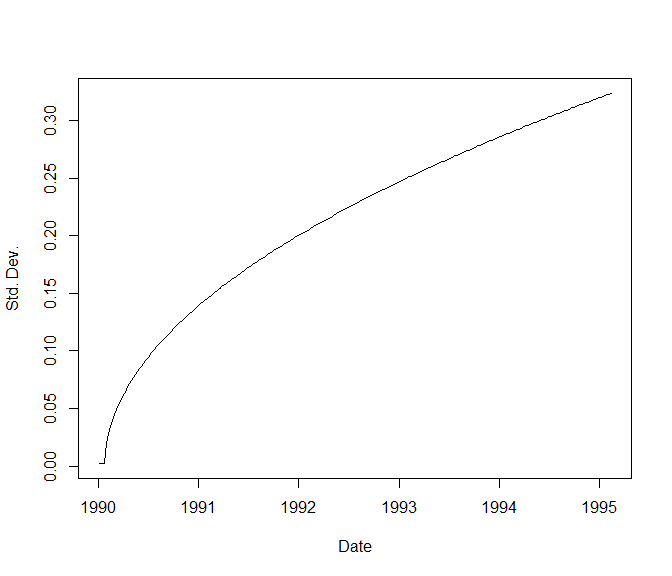Due to the time homogeneous time to maturity of the stitched contract data, the variance of the first state variable is linear through time, with the standard deviation having a square-root relationship through time. plot(Observation.Dates, sqrt(SS.Oil.2F$P_t[2,2,]), type = 'l',
xlab = "Date", ylab = "Std. Dev.")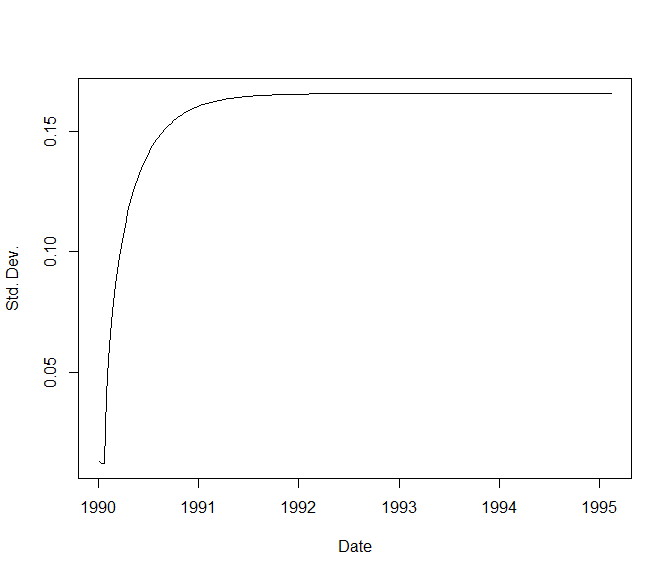Due to the time homogeneous time to maturity of the stitched contract data, the standard deviation of the second state variable approaches a steady state distribution.

plot(as.Date(rownames(SS.Oil$Contracts)), sqrt(SS.Oil.2F.Contracts$P_t[1,1,]),
type = 'l', xlab = "Date", ylab = "Std. Dev.",
main = "Time Series of the Std. Dev for State Variable 1")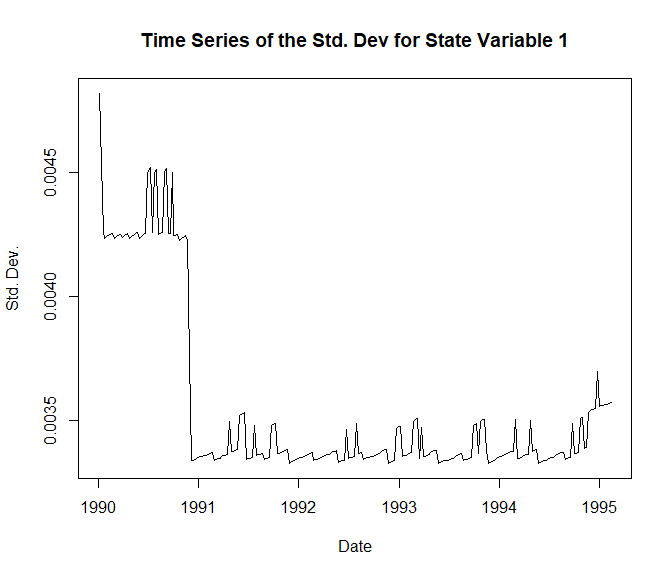A possible structural break at approximately the beginning of 1991 can be observed within the plot above. In the work of Cortazar and Naranjo (2006), structural breaks in time series data were tested by comparing the time-series covariance matrix of state variables for difference samples, and then computing a Wald test statistic.

plot(x = Observation.Dates, sqrt(SS.Oil.2F.Contracts$P_t[2,2,]), type = 'l', xlab = "Date", ylab = "Std. Dev.", main = "Time Series of the Std. Dev for State Variable 2")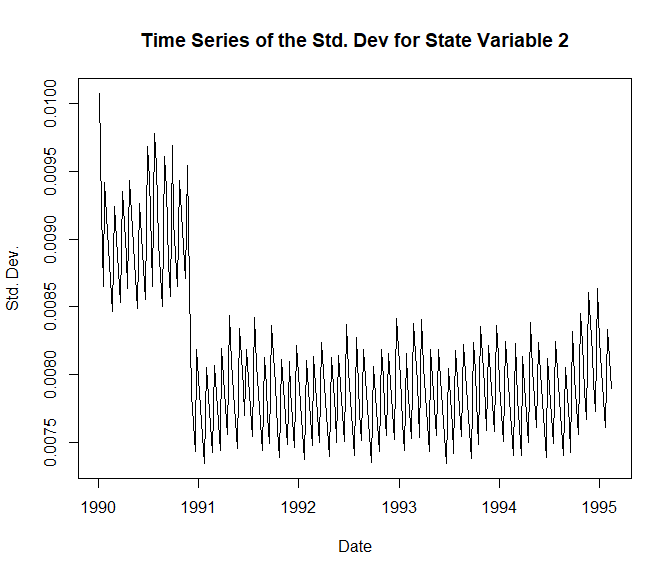Factor two follows an Ornstein-Uhlenbeck process and the mean-reverting behavior of this state variable is clearly shown in the plot above ### 4.9 Forecast Spot and Futures Prices: Expected Spot and futures prices can be forecast under an N-factor model analytically through the ‘Spot.Price.Forecast’ and ‘Futures.Price.Forecast’ functions. ‘help(Spot.Price.Forecast)’ and ‘help(Futures.Price.Forecast)’ presents expressions of expected spot and futures prices respectively. Forecasted spot and futures prices were presented in the work of Schwartz and Smith (2000) in figures 1 and 2 using parameters developed using Enron data. ##Figure 1 and 2 of Schwartz and Smith (2000) was developed using Enron data ##and an assumption that mu was approximately 3% p.a.: Enron.values <- c(0.0300875, 0.0161, 0.014, 1.19, 0.115, 0.158, 0.189) names(Enron.values) <- NFCP.Parameters(2, TRUE, FALSE, FALSE, 0, FALSE) #### 4.9.1 Spot Prices: The work of Schwartz and Smith (2000) presented forecasts of spot prices developed using Enron data (figure 1): ## Replicate figure 1 of Schwartz and Smith (2000): SS.Expected.Spot <- Spot.Price.Forecast(X.0 = c(2.857, 0.119), Enron.values, t = seq(0,9,1/12), Percentiles = c(0.1, 0.9)) ##Factor one only: Equilibrium.theta <- Enron.values[!names(Enron.values) %in% c("kappa_2", "lambda_2", "sigma_2", "rho_1_2")] SS.Expected.Equilibrium <- Spot.Price.Forecast(X.0 = c(2.857, 0), Equilibrium.theta, t = seq(0,9,1/12), Percentiles = c(0.1, 0.9)) SS.Figure.1 <- cbind(SS.Expected.Spot, SS.Expected.Equilibrium) matplot(seq(0,9,1/12), SS.Figure.1, type = 'l', col = 1, lty = c(rep(1,3), rep(2,3)), xlab = "Time (Years)", ylab = "Spot Price ($/bbl, WTI)",
main = "Probabilistic Forecasts for Spot and Equilibrium Prices")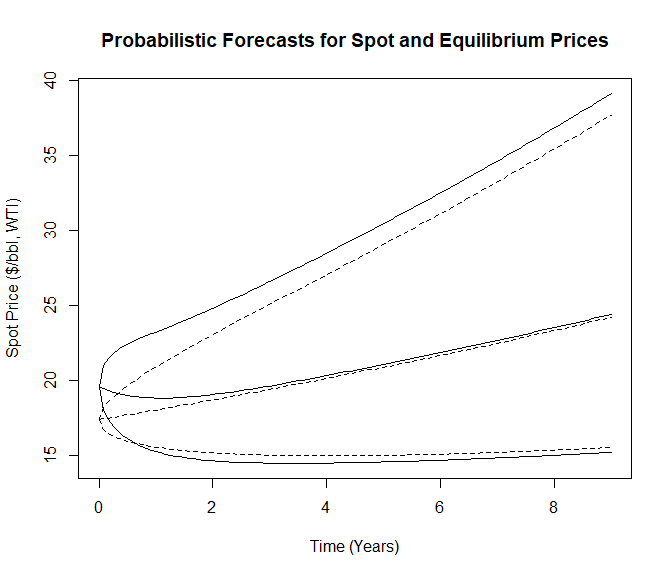An important consideration when forecasting spot prices using parameters estimated through MLE is that the parameter estimation process takes the assumption of risk-neutrality and thus the true process growth rate $$\mu$$ is not estimated with a high level of precision. For example, the standard error of parameter $$\mu$$ for the one-factor GBM estimated in section 4.3 was approximately 10%. This is further shown such that Schwartz and Smith (2000) did not use the estimated value of $$\mu$$ to forecast spot prices, opting to set it at a value “that is more representative of investor expectations” (Schwartz and Smith (2000)).

#### 4.9.2 Futures Prices

Risk-neutral parameters are directly observed in the parameter estimation process and are therefore estimated with a higher level of precision.

Futures prices of Enron data was presented in the work of Schwartz and Smith (2000) in figure 2:

## Replicate Figure 2 of Schwartz and Smith (2000):

#Forecast expected spot prices under the "True" stochastic process:
SS.Expected.Spot <- Spot.Price.Forecast(X.0 = c(2.857, 0.119),
parameters = Enron.values,
t = seq(0,9,1/12),
Percentiles = c(0.1, 0.9))
#Forecast expected futures prices under the Risk-Neutral stochastic process:
SS.Futures.Curve <- Futures.Price.Forecast(X.0 = c(2.857, 0.119),
parameters = Enron.values,
TTM = seq(0,9,1/12))
SS.Figure.2 <- cbind(SS.Expected.Spot[,2], SS.Futures.Curve)
matplot(seq(0,9,1/12), log(SS.Figure.2), type = 'l', col = 1,
xlab = "Time (Years)", ylab = "Log(Price)",
main = "Futures Prices and Expected Spot Prices")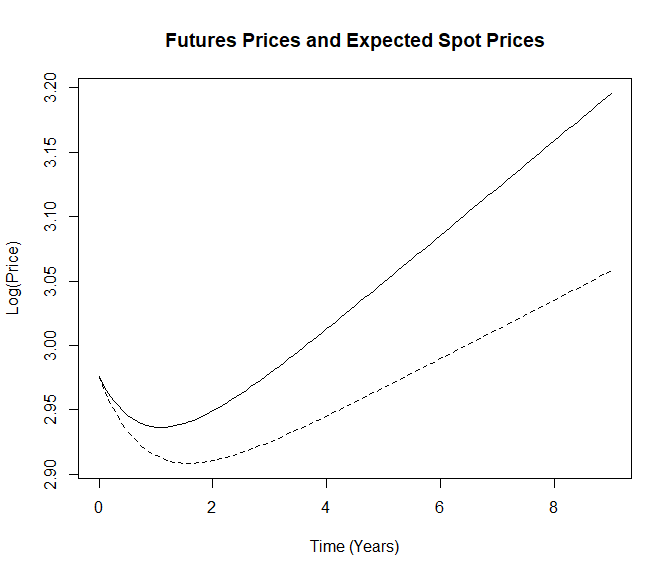#### 4.9.3 Plot the Futures Curve:

The model fit to the observed term structure on given dates can be used as a measure of robustness of N-factor commodity pricing models. This also allows for analysis to how the model fits under circumstances of strong backwardation or contango, exogenous shocks, etc.

The futures curve can be forecast on a particular date using the estimate state vector and compared to the observed futures prices at that time. For example, the fit of the estimated one-factor GBM model and the two-factor model of Schwartz and Smith (2000) can be plotted against observed oil futures prices on the final observation date:

## Maximum Observed Maturity:
Max.Maturity <- max(tail(SS.Oil$Contract.Maturities,1), na.rm = TRUE) ##Estimated Futures Prices: ### One Factor (GBM): Oil.TS.1F <- Futures.Price.Forecast(X.0 = SS.Oil.1F$X.t,
parameters = SS.Oil.1F$Estimated.Parameters, TTM = seq(0,Max.Maturity,1/12)) ### Two Factor: Oil.TS.2F <- Futures.Price.Forecast(X.0 = SS.Oil.2F$X.t,
parameters = SS.Oil$Two.Factor, TTM = seq(0,Max.Maturity,1/12)) matplot(seq(0,Max.Maturity,1/12), cbind(Oil.TS.1F, Oil.TS.2F), type = 'l', xlab = "Maturity (Years)", ylab = "Futures Price ($)",
main = "Estimated and observed oil futures prices on 1995-02-14");
points(tail(SS.Oil$Contract.Maturities,1), tail(SS.Oil$Contracts,1))
legend("bottomleft", c("One-factor", "Two-Factor", "Observed"),
col=2:4,cex=0.8,fill=c(1,2,0))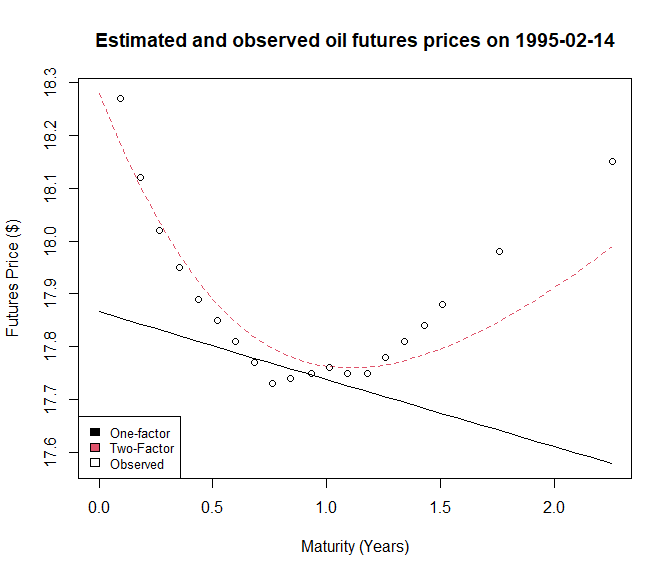The additional flexibility of the two-factor model better fits the observed term structure at this particular observation date, particularly because the one-factor GBM model is linear in forecasts, which does not describe the observed behavior of futures prices. Comparing the models ability across different observation dates and when the market is in different levels of backwardation, contango, etc. can be used as a measure of robustness and fit of commodity pricing models.

### 4.10 Plot Volatility Term Structure:

In the work of Cortazar and Naranjo (2006), the ability of an N-factor model to match the volatility term structure of futures returns is presented as a measure of robustness (figure 7). This robustness measure can be attained by calling the ‘TSFit.Volatility’ function, but it is also returned by the ‘NFCP.Kalman.filter’ function when ‘verbose = TRUE’.

###Test the Volatility Term Structure Fit of the Schwartz-Smith Two-Factor Oil Model:
V_TSFit <- TSFit.Volatility(
parameters = SS.Oil$Two.Factor, Futures = SS.Oil$Stitched.Futures,
TTM = SS.Oil$Stitched.TTM, dt = SS.Oil$dt)

##Plot Results:
matplot(V_TSFit["Maturity",], cbind(SS.Oil.1F$TSFit.Volatility["Theoretical Volatility",], V_TSFit["Theoretical Volatility",]), type = 'l', xlab = "Maturity (Years)", ylab = "Volatility (%)", ylim = c(0, 0.5), main = "Volatility Term Structure of Futures Returns"); points( V_TSFit["Maturity",], V_TSFit["Empirical Volatility",]); legend("bottomleft", c("Empirical", "One-Factor", "Two-Factor"),col=0:2,cex=0.8,fill=0:2)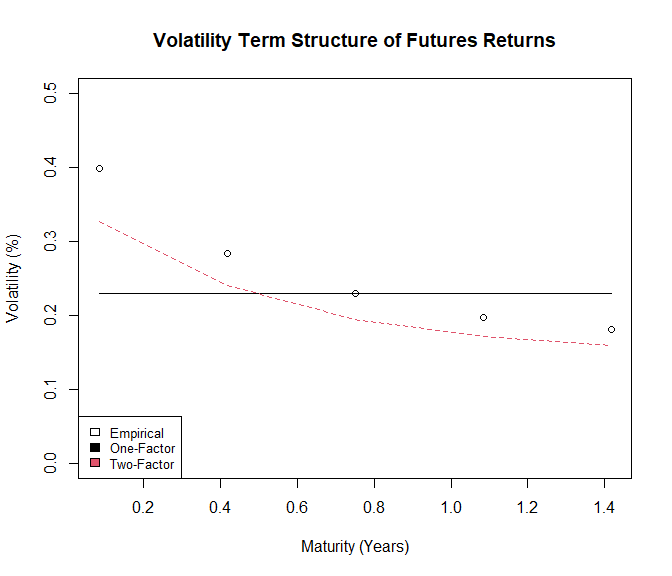This finding shows that the two-factor model tends to slightly underestimate the volatility term structure of futures returns of this dataset. The two-factor model has a better fit to the volatility term structure over the one-factor GBM model, however, as it considers volatility to be a depreciating function with respect to the time to maturity of a futures contract. Additional factors could possibly have a better fit to this robustness measure. A larger number of factors provides the model with more flexibility in adjusting first and second moments simultaneously, possibly explaining the ability for models with more factors to better describe the volatility term structure of futures returns. ### 4.11 Simulate Spot and Futures Prices: Monte Carlo simulation can be used to numerically simulate future price paths of spot and futures prices under an N-factor model through the ‘Spot.Price.Simulate’ and ‘Futures.Price.Simulate’ functions. Valuation of American options under an N-factor model generally requires Monte Carlo methods due to the multidimensional structure of N-factor models. The simulation of future price paths is therefore of great interest to those evaluating American options, investment under commodity price uncertainty, and Real Options Analysis. The solutions of simulating spot and futures prices are presented withing ‘help(Spot.Price.Simulate)’ and ‘help(Futures.Price.Simulate)’. #### 4.11.1 Spot Prices: Spot prices are simulated using the ‘mvrnorm’ function of the ‘MASS’ package to quickly and efficiently estimate futures shocks of price paths. 100 simulations with a forecasting horizon of 1 year and a monthly (ie. 1/12) discrete time step can be computed through the following: ##100 antithetic simulations of one year of monthly observations Simulated.Spot.Prices <- Spot.Price.Simulate( X.0 = SS.Oil.2F$X.t,
parameters = SS.Oil$Two.Factor, t = 1, dt = 1/12, n = 1e3, antithetic = TRUE, verbose = TRUE) ##Plot Price Paths: matplot(seq(0,1,1/12), Simulated.Spot.Prices$Prices, type = 'l',
xlab = "Forecasting Horizon (Years)",
ylab = "Spot Price ($/bbl, WTI)", main = "Simulated Crude Oil prices")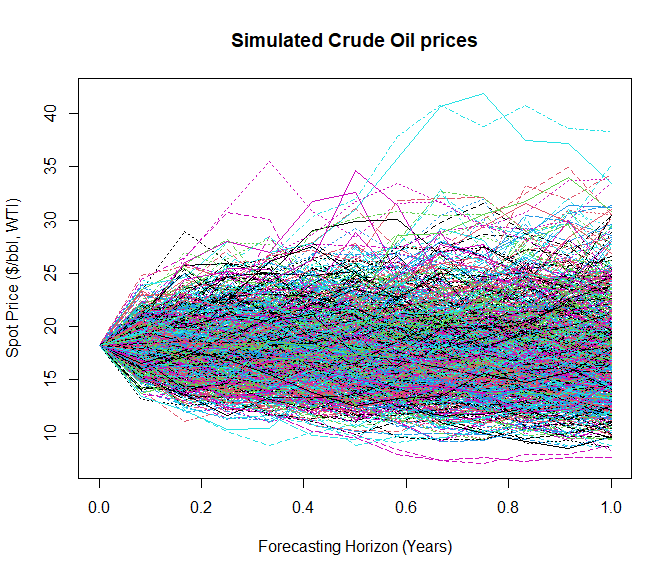Spot prices are simulated using antithetic price paths by default, a simple and common variance reduction technique: ##Not Run - Plot Antithetic Price Pairs: matplot(seq(0,1,1/12), Simulated.Spot.Prices$Prices[,1:2], type = 'l',
xlab = "Forecasting Horizon (Years)",
ylab = "Spot Price ($/bbl, WTI)", main = "Simulated Crude Oil Antithetic Price Pair")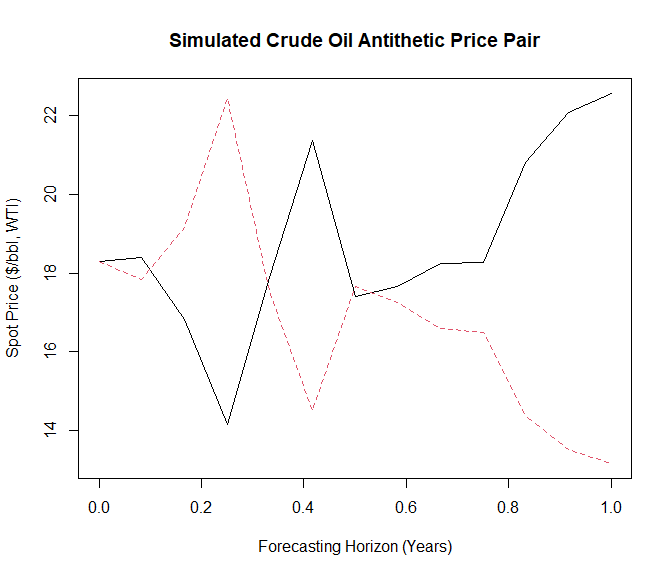Confidence intervals of simulations can also be visualized: ##Plot 95% Prediction interval: Prediction.interval <- rbind.data.frame(apply(Simulated.Spot.Prices$Prices, 1,
FUN = function(x) stats::quantile(x, probs = c(0.025, 0.975))),
Mean = rowMeans(Simulated.Spot.Prices$Prices)) matplot(seq(0,1,1/12), t(Prediction.interval), type = 'l', col = c(2,2,1), lwd = 2, lty = c(2,2,1), xlab = "Forecasting Horizon (Years)", ylab = "Spot Price ($/bbl, WTI)", main = "Simulated Crude Oil 95% Confidence Interval")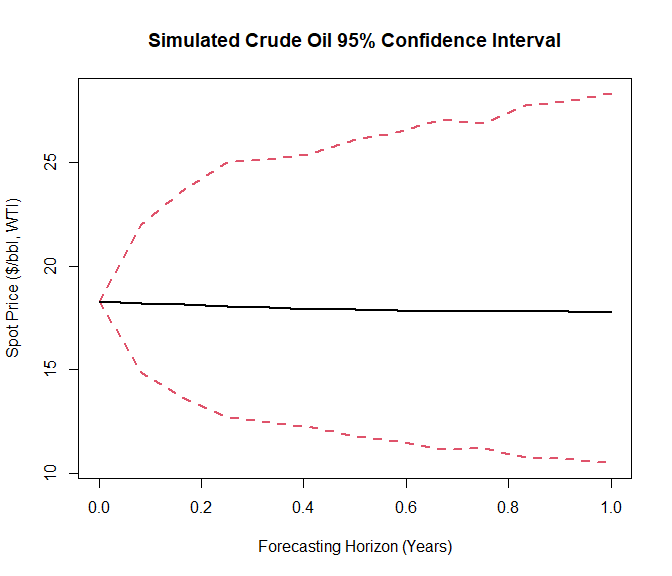#### 4.11.2 Futures Prices:

Aggregated, stitched futures data as well as individual contracts are able to be simulated through the ‘Futures.Price.Simulate’ function:

## Simulate Crude Oil Contract Prices under a Two-Factor model

Simulated.Contracts <- Futures.Price.Simulate(X.0 = c(log(SS.Oil$Spot[1,1]), 0), parameters = SS.Oil.Two.Factor, dt = SS.Oil$dt,
N.obs = nrow(SS.Oil$Contracts), TTM = SS.Oil$Contract.Maturities)

##Not Run - plot Simulated prices:
matplot(as.Date(rownames(SS.Oil$Contracts)), Simulated.Contracts$Futures,
type = 'l', ylab = "Futures Price ($/bbl, WTI)", xlab = "Observations", main = "Simulated Futures Contracts")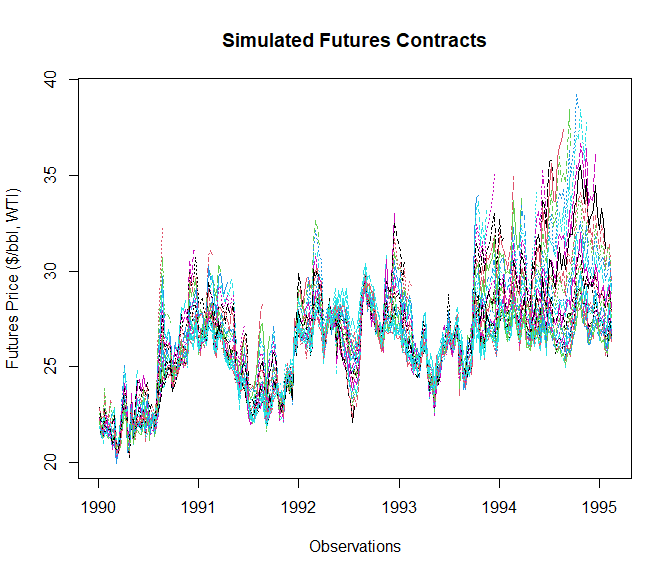Simulating futures prices can be useful for various analytic purposes, including the ability for the parameter estimation procedure to find the ‘true’ parameters of the spot price process that futures prices were simulated from. ### 4.12 Option Valuation: The primary purpose of commodity pricing models is for the valuation and hedging of commodity contingent claims (i.e. derivative products on the commodity). Under the N-factor framework, European call and put options can be valued analytically through the Black and Scholes stock-option model applied to the N-factor framework. Schwartz and Smith (2000) first presented analytic European option pricing under a two-factor model. The value of American put options can also be approximated numerically under an N-factor framework through the least-squares Monte-Carlo (LSM) simulation approach. The LSM simulation method is an option valuation method first presented by Longstaff and Schwartz (2001) that approximates the value of American options by using “least squares to (directly) estimate the conditional expected payoff to the option holder from continuation” (Longstaff and Schwartz, 2001). The following section presents the valuation of European and American put options under the N-factor framework, considering and comparing the value of options under both a one-factor and two-factor commodity pricing model. #### 4.12.1 European Call and Put Options: European call and put options can be valued analytically under an N-factor model through the ‘European.Option.Value’ function. Expressions of the value of European call and puts under an N-factor model are presented in ‘help(European.Option.Value)’. The final filtered values of the state variables is generally used to forecast future prices and value European options. These can be obtained by the ‘X.t’ object returned within the list object of ‘NFCP.Kalman.filter’ function when argument ‘verbose = TRUE’. Consider a European put option on the spot price of crude oil, with option expiry in 1 year and a strike price of$20/bbl. Assume the risk-free interest rate is 5%. The calculated option value under the one-factor model estimated in section 4.3 and the two-factor model of Schwartz and Smith (2000) are:

## One-Factor European put option value:
European.Oil.1F <- European.Option.Value(X.0 = SS.Oil.1F$X.t, parameters = SS.Oil.1F$Estimated.Parameters,
t = 1,
TTM = 1,
K = 20,
r = 0.05,
call = FALSE)

## Two-Factor European put option value:
European.Oil.2F <- European.Option.Value(X.0 = SS.Oil.2F$X.t, parameters = SS.Oil$Two.Factor,
t = 1,
TTM = 1,
K = 20,
r = 0.05,
call = FALSE)
## Print results:
print(round(c(One.Factor = European.Oil.1F, Two.Factor = European.Oil.2F),3))
#> One.Factor Two.Factor
#>      2.936      2.187

Option values under different stochastic modeling assumptions and number of factors can vary greatly. When argument ‘verbose = TRUE’, the ‘European.Option.Value’ function returns a list object providing details of the sensitivity in option price to the different stochastic parameters of the N-factor model as well as numeric approximations of the most common ‘Greeks’ considered in European option pricing.

#### 4.12.2 American Put Options:

The valuation of American put options under the N-factor framework is available through the ‘American.Option.Value’ function. ‘American.Option.Value’ is a wrapper to the LSM simulation implementation ‘LSM.AmericanOption’ of the ‘LSMRealOptions’ package (Aspinall et al., 2021). LSM simulation is one of the most popular methods of approximating the value of American-style options due to its ability to efficiently and easily handle multi-dimensional valuating settings. This property of LSM simulation makes it the ideal solution method to value American-style options under the N-factor framework.

Longstaff and Schwartz (2001) state that as the conditional expectation of the continuation value belongs to a Hilbert space, it can be represented by a combination of orthogonal basis functions, where increasing the number of stochastic state variables (i.e. factors) increases the number of required basis functions exponentially. The convergence properties of the LSM simulation function state that the American option value calculated by the LSM simulation method converges to the true value as the number of simulations and basis functions increase (Longstaff and Schwartz, 2001; Clément, Lamberton and Protter, 2002). LSM simulation can be a computationally expensive process, with processing times increasing as a function of the number of simulations used and the degree of the orthogonal polynomial that is applied.

Similarly to the European option valuation presented in 4.12.1, Consider an American put option on the spot price of crude oil, with option expiry in 1 year and a strike price of $20/bbl. Assume the risk-free interest rate is 5%. Furthermore, consider 100,000 simulations (of which 50% are antithetic) and an exercise opportunity of 50 times per year. The calculated option value under the one-factor model estimated in section 4.3 and the two-factor model of Schwartz and Smith (2000) are: ## One-Factor American put option value: American.Oil.1F <- American.Option.Value(X.0 = SS.Oil.1F$X.t,
parameters = SS.Oil.1F$Estimated.Parameters, n = 1e5, t = 1, dt = 1/50, K = 20, r = 0.05) ## Two-Factor American put option value: American.Oil.2F <- American.Option.Value(X.0 = SS.Oil.2F$X.t,
parameters = SS.Oil$Two.Factor, n = 1e5, t = 1, dt = 1/50, K = 20, r = 0.05, verbose = FALSE, orthogonal = "Power", degree = 2) print(round(c(One.Factor = American.Oil.1F, Two.Factor = American.Oil.2F),3)) #> One.Factor Two.Factor #> 2.956 3.697 The ability to exercise options at any point before maturity is a premium that American-style options hold over European-style options and therefore the value of American-style options are always greater than European-style counterparts. #### 4.12.3 Replicate table 1 of Longstaff and Schwartz (2001): Longstaff and Schwartz (2001) present a table (table 1) of calculated vanilla American put option values under differing levels of strike prices, annual volatility of the underlying asset and option maturity. Corresponding analytic European and simulated American option values can be replicated through the ‘European.Option.Value’ and ‘American.Option.Value’ functions respectively. Following the work of Longstaff and Schwartz (2001), consider an American-style put option on a share of stock that can be exercised at 50 discrete time points per year. The strike price of the put is$40, the short-term interest rate is .06. American options are valued using the LSM simulation solution method, using 100,000 simulations (of which 50% are antithetic) of price paths of the stock-price process, which is assumed to be a GBM stochastic process.

## Exercise opportunities per year:
dt <- 1/50
## strike price :
K <- 40
## short-term interest rate:
rf <- 0.06
## 100,000 simulations (50% antithetic):
N.simulations <- 1e5
## Stock price volatility (variable):
sigma <- rep(c(rep(0.2,2),rep(0.4,2)),5)
## Initial Stock price (variable):
S0 <- sort(rep(seq(36,44,2),4))
## Option maturity (variable):
TTM <- rep(1:2, 10)

LSM.output <- matrix(0, 20, 4,
dimnames = list(NULL, c("LSM American", "(s.e)",
"Closed form European",
"Early exercise value")))

## Cycle through the rows of the table:
for(i in 1:20){

## Stochastic model assumption:
Stockparameters <- c(mu_star = (rf - 0.5 * sigma[i]^2), sigma_1 = sigma[i])

## American option pricing through LSM Simulation
output <- American.Option.Value(X.0 = log(S0[i]),
parameters = Stockparameters,
n = N.simulations,
t = TTM[i],
dt = dt,
K = K,
r = rf,
orthogonal = "Laguerre",
degree = 3,
verbose = TRUE)

LSM.output[i,1] <- output$Value LSM.output[i,2] <- output$Standard Error

## European option pricing through the BSM PDE
LSM.output[i,3] <- European.Option.Value(X.0 = log(S0[i]), parameters = Stockparameters,
t = TTM[i], TTM = TTM[i], K = K, r = rf, call = FALSE)

## Early exercise value - the difference between American and European put values:
LSM.output[i,4] <- LSM.output[i,1] - LSM.output[i,3]

}

The first three degree’s of the “Laguerre” orthogonal polynomial are used in this example, as “three basis functions are sufficient to obtain effective convergence of the algorithm in this example” (Longstaff and Schwartz, 2001).

## Compile and print results:
LnS.Table1 <- cbind.data.frame(S = S0, sigma = sigma, T = TTM, LSM.output)
print(round(LnS.Table1,3))
#>     S sigma T LSM American (s.e) Closed form European Early exercise value
#> 1  36   0.2 1        4.471 0.009                3.844                0.627
#> 2  36   0.2 2        4.829 0.011                3.763                1.066
#> 3  36   0.4 1        7.096 0.019                6.711                0.384
#> 4  36   0.4 2        8.487 0.022                7.700                0.787
#> 5  38   0.2 1        3.243 0.009                2.852                0.391
#> 6  38   0.2 2        3.742 0.011                2.991                0.752
#> 7  38   0.4 1        6.140 0.019                5.834                0.306
#> 8  38   0.4 2        7.650 0.022                6.979                0.671
#> 9  40   0.2 1        2.313 0.009                2.066                0.247
#> 10 40   0.2 2        2.873 0.011                2.356                0.517
#> 11 40   0.4 1        5.301 0.018                5.060                0.242
#> 12 40   0.4 2        6.899 0.021                6.326                0.573
#> 13 42   0.2 1        1.622 0.008                1.465                0.157
#> 14 42   0.2 2        2.202 0.010                1.841                0.361
#> 15 42   0.4 1        4.567 0.017                4.379                0.188
#> 16 42   0.4 2        6.218 0.021                5.736                0.482
#> 17 44   0.2 1        1.111 0.007                1.017                0.094
#> 18 44   0.2 2        1.694 0.009                1.429                0.265
#> 19 44   0.4 1        3.951 0.016                3.783                0.168
#> 20 44   0.4 2        5.611 0.020                5.202                0.409

The ‘LSMRealOptions’ provides a framework for the calculation of American-style option values, including the valuation of capital investment projects, through the LSM simulation method. ‘LSMRealOptions’ natively supports the simulated factors output by the ‘Spot.Price.Simulate’ and is appropriate for other derivative pricing of commodity contingent claims.

# 5 Discussion:

This work has presented the ‘NFCP’ (N-Factor Commodity Pricing) package, providing worked examples of the functionality of the package, replicating the work of Schwartz and Smith (2000) throughout the study. The ‘NFCP’ package is a very versatile package that provides the ability to effectively build, analyse, forecast and simulate commodity pricing models. The ‘NFCP’ package allows for a wide versatility in the structure of commodity pricing models, allowing the spot price process of the commodity to be made up of the exponential sum of N arbitrary factors, with both random walk and mean-reverting characteristics available. The ‘NFCP’ package combines the fastest Kalman filter algorithm available with an effective optimization algorithm to ensure MLE returns optimal parameters for a given commodity pricing model and term structure data. The numeric difficulty of parameter estimation increases sharply with the number of underlying factors, N. The number of unknown parameters of an N-factor model (without considering the white noise of futures contracts) is equal to: $$0.5 (N^2 + 5N)$$. Realistically, commodity pricing models with more than four factors are impractical due to the difficulty of parameter estimation through MLE.

The parameter estimation process is a bounded search under which the parameters can only take realistic values (i.e. volatility terms cannot be negative, etc.). Parameters estimated through MLE, however, have no in-built guarantee that they will make explicit economic sense. Output analysis of MLE is a crucial step in ensuring commodity pricing models are appropriate for analysis purposes. Factors with extremely large estimated correlation coefficients (say, greater than 90% or less than -90%) are generally poorly specified, with the commodity pricing model failing to differentiate between the dynamics that drive these two factors. Mean-reverting factors with extremely large risk-premium parameters ,$$\lambda_i$$, are considered poorly specified models and generally include factors with very high correlation coefficients. Finally, reversion rates, $$\kappa_i$$, that are extremely high or low are a final indicator of poorly specified models. A reversion rate that is too low is representative of a factor that does not revert over time, thus inducing a unit root in the spot price process. If this is encountered when GBM = FALSE, this may be the model naturally inducing a unit root, indicating that a degree of the spot price process is driven by a random walk. If this is encountered when GBM = TRUE, it’s not appropriate to utilize the commodity pricing model under the assumption that spot prices are driven by more than one unit-root process. When mean-reversion rates are extremely high, the state variable approaches a deterministic value given by the equilibrium of the process. Although log-likelihood scores for some commodity pricing models may indicate their increased ability to explain the term structure of a commodity, output analysis of parameters estimated through MLE is a crucial step to evaluating the applicability of estimated models for forecasting and analysis purposes.

The ‘NFCP’ package is a comprehensive and powerful framework for the development and analysis of commodity pricing models. It provides the ability to forecast and value derivative products using existing term structure data, and further provides the tools required to value American option products through simulation methods. The development and analysis of commodity pricing models is highly recommended to those who interact and trade in commodity markets, or those who have investment projects with the returns of these project contingent on the prices of commodities.